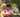### Distinct

January 17, 2020

Distinct

Write a function
function solution(A);
that, given an array A consisting of N integers, returns the number of distinct values in array A.

For example, given array A consisting of six elements such that:
A = 2
A = 1
A = 1
A = 2
A = 3
A = 1
the function should return 3, because there are 3 distinct values appearing in array A, namely 1, 2 and 3.

Write an efficient algorithm for the following assumptions:
• N is an integer within the range [0..100,000];
• each element of array A is an integer within the range [−1,000,000..1,000,000].

Use Set() to store unique values.

``````function solution(A) {
// write your code in JavaScript (Node.js 8.9.4)
const dataset = new Set()